# Electronics and Communication Engineering - Exam Questions Papers

31.

A transmitted using AM has in modulated carrier output power of 10 KW and can be modulated to a maximum depth of 90% by a sinusoidal modulating voltage without causing overloading. Find the value to which an modulated carrier power can be increased without resulting in overloading if the maximum permitted modulating index is 40%

 A. 14 KW B. 12.96 KW C. 26.96 KW D. 2.96 KW

Explanation: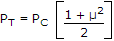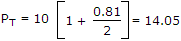Now PT is fixed, μ = 0.4

Then PC is PC =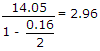32.

The signal flow graph of a system is shown in figure. The transfer function of the system is :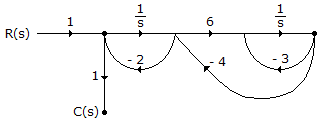A.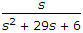B.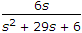C.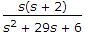D.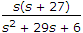Explanation:

P1 = 1 [Path R(s to C(s)]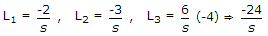Δ = 1 - (L1 - L2 + L3) + L1L2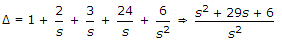P1 = 1; Δ1 = 1

[Non touching loop gain to forward path]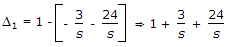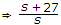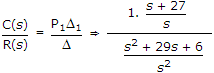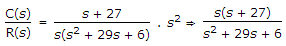.

33.

The Laplace transform of the given waveform is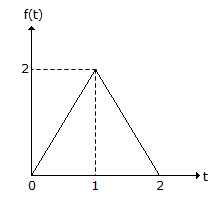A.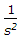(1 - e-s + e-2s) B.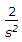(1 - e-s + e-2s) C.(1 - 2e-s + e-2s) D.(1 - 2e-s)

Explanation: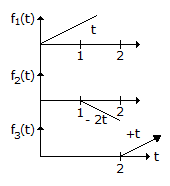f(t) = f1(t) + f2(t) + f3(t)

= 2tu(t) - 2 x 2(t - 1) + 2(t - 2)u(t - 2)

f(s) =-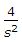e-s +e-2s

=(1 - 2e-s + e-2s).

34.

With respect to the following 555 timer circuit what will be the value of RA and R such that the LED turns on for a duration of 10 ms every time it receives negative trigger pulse. LED operating current = 20 mA, Vf = 1.4 V, Vo on = 13.4 V and C = 0.22 nf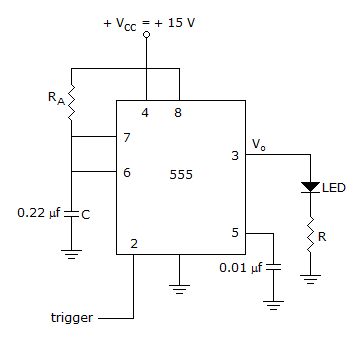A. 41.4 KΩ, 600 Ω B. 45 KΩ, 630 Ω C. 43.5 KΩ, 580 Ω D. 44.9 KΩ, 600 Ω

Explanation:

Timer operated in mono, mode

RAC =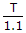RA = 41.4 KΩ

R =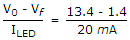= 600 Ω.

35.

The region between a pin of parallel perfectly conducting planes of infinite extent is y and z directions is partially filled with a dielectric as shown below.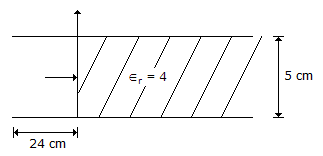A 30 GHz TE10 wave is incident on the air-dielectric interface. The value of reflection coefficient at the interface is

 A.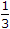B.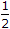C.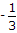D.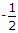Explanation: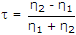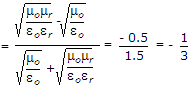#### Current Affairs 2022

Interview Questions and Answers